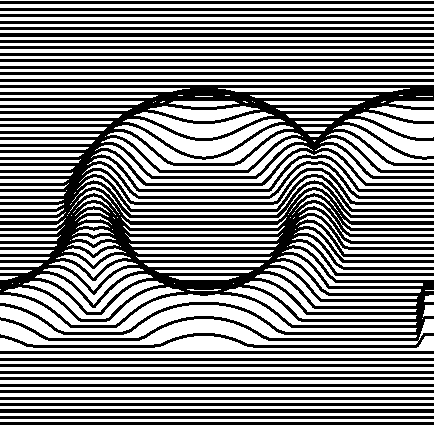Topographic Form

Jay Kominek

Based on: Topographic Form by Sture Johannesson, 1976

Category: direct

Description:

This sketch does not run in the browser./*
Part of the ReCode Project (http://recodeproject.com)
Based on "Topographic Form" by Sture Johannesson
Originally published in "Computer Graphics and Art" v1n2, 1976
*/

/* @pjs pauseOnBlur="true"; */

/*
Part of the ReCode Project (http://recodeproject.com)
Based on "Topographic Form" by Sture Johannesson
Originally published in "Computer Graphics and Art" vol1 no2, 1976
*/

// by Sture Johannesson and Sten Kallin, Malmo, Sweden

class RaisedArc {
float cx, cy;
float r;
float width;
float start, stop;
float height;
RaisedArc(float x_, float y_,
float r_, float width_,
float start_, float stop_,
float height_)
{
cx = x_; cy = y_; r = r_;
width = width_/2.0;   height = height_;
start = start_;   stop = stop_;
}

// the arc is effectively -pi/2 to pi/2 of cosine,
// stretched to the specified width and height, and extruded
// along the arc centered at x,y and r units from there,
float computeHeight(float px, float py)
{
float rx = px - cx, ry = py - cy;

float theta = atan2(ry, rx) + PI;
float distance = sqrt(rx*rx + ry*ry);

if( (start <= theta) && (theta <= stop) )
{
float wp = abs(r - distance) / width;

// you can comment this out to get "ringing"
// from the arcs.
if(wp >= 1.0) wp = 1.0;

return height * cos(PI/2.0 * wp);
}
else
return 0.0;
}
}

RaisedArc[] arcs;
int sh = 600;
int sw = 3*(sh/2);

// you can make a cleaner looking version by permitting smoothing,
// and making the fx step much, much smaller.
// decreasing the fx step eliminates the slight appearance of perspective at
// the ends of the partial arcs, though. (you can see evidence of large fx
// steps in the original about halfway up the outside of the left arc.)

void setup()
{
arcs = new RaisedArc;
arcs = new RaisedArc(  0, 0, 15, 9, 0, 2*PI, 10);
arcs = new RaisedArc(-30, 0, 15, 9, PI, 2*PI, 10);
arcs = new RaisedArc(-30, 0, 15, 9, 0, PI/2, 10);
arcs = new RaisedArc( 30, 0, 15, 9, 0, 1.5*PI, 10);
size(sw, sh, P3D);
noSmooth();
noFill();
strokeWeight(0.8);
}

void draw()
{
// black on white
background(255);
stroke(0);

// the XY plane is tilted, but it is hard to tell
float rotation = 28.12 / 180.0 * PI;
// because the projection is orthographic
ortho();

// scoot us to the middle
translate(sw/2, sh/2, 0);
// scale things up. values made up to fit the window.
scale((sw/2)/60*1.05, (sh/2)/41*1.05, 1.0);

rotate(rotation, 1, 0, 0);

beginShape();
for(int iy = -41; iy < 41; iy++)
{
float fy = (float)iy;

// this flip flops the direction in which we're drawing. no way to know
// for sure from the original, but it would work better with any sort of
// scanning display device (storage scopes, etc), and simplifies the little
// connections at the end.
float fx_start = ((iy%2)!=0)?-60.0:60.0;
float fx_stop  = -fx_start;
float fx_step  = ((iy%2)!=0)?1.0:-1.0;
for(float fx = fx_start; ((iy%2)!=0)?(fx<=fx_stop):(fx>=fx_stop); fx += fx_step)
{
float fz = 0.0;

// height of our surface is the height of the tallest arc at that spot.
for(int j=0; j<4; j++)
{
float h = arcs[j].computeHeight(fx, fy-0.5);
if(h > fz)
fz = h;
}

// emit a vertex. i bet this part was more complicated in the original.
vertex(fx,fy,fz);
}
}
endShape();
}